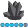## Another 'Invalid numeric data' question

Hi:

I know there has already been a lot of questions and answers about the ' invalid numeric data error' out there, but after browsing it seems that I can't find one similar to the problem that I encounter. I have a bunch of very similar character variables (e.g. study1 - study 40), and I am trying to use a do loop together with array to recode the 40 variables into 40 new variables called type1-type40. Here is my code:

data want;

set have;

array experiment{40} study1-study40;

array type {40};

do i=1 to 40;

if experiment{i} in ('106','112','123','133','135','139'')then type{i}=1;

else if experiment{i} in ('103 - PartA','103 - PartB', '109 - PartA','109 - PartB','126 - PartA','126 - PartB') then type{i}=2;

else if experiment{i}=. then type{i}=.;

else if experiment{i}='Ethics train' then type{i}=.;

else type{i}=0;

end;

run;

Here is the error message that I keep getting:

NOTE: Invalid numeric data, 'Ethics train' , at line 1397 column 13.

I have double checked using proc contents, that all study1-study40 variables are characters. I don't know where the error message comes from.

Thank you very much!

Cici

2 REPLIES 2Jim_G
Pyrite | Level 9

## Re: Another 'Invalid numeric data' question

Define your experiment array as character with a \$10 in the statement .
Then your else if compare experiment{i} to one blank ' ' not missing (.)MikeZdeb
Rhodochrosite | Level 12

## Re: Another 'Invalid numeric data' question

Hi, since you have the ARRAY statement after the SET statement, you should not have to explicitly state that the array EXPERIMENT is CHARACTER (it's implicitly known at that point given that the STUDY variables are character).

One thing that might be causing a problem is this line ...

if experiment{i} in ('106','112','123','133','135','139'')then type{i}=1;

You have an extra QUOTATION MARK at the end of the value list in the IN clause.

Also, this should cause a comment in the LOG about variable type conversion ...

else if experiment{i}=. then type{i}=.;

EXPERIMENT is a CHARACTER array, so that period for a missing value should be a space (" ") or just use ...

else if missing(experiment{i}) then type{i}=.;

and you don't have to worry about variable type.

A suggestion, how about using a FORMAT to recode you varaible values ...

proc format;
value \$e2t
'106','112','123','133','135','139' = '1'
'103 - PartA','103 - PartB', '109 - PartA','109 - PartB',

'126 - PartA','126 - PartB' = '2'
'Ethics train', ' ' = ' '
other = '0'
;
run;

data have;
infile datalines dsd;
input study1 :\$15. @@;
study2 = study1;
datalines;
'106', '112', '123', '133', ,'135','139'
'103 - PartA', '103 - PartB', '109 - PartA', '109 - PartB'

'126 - PartA', '126 - PartB'
'Ethics train', ' ', '999999999'
;

data want (drop=j);
set have;
array experiment(2) study: ;
array topic(2);
do j=1 to 2;

* use the INPUT function to make TOPIC a numeric variable;
topic(j) = input(put(experiment(j),\$e2t.),1.);
end;
run;

If you print data set WANT you'll see that all the recoding works with a format.

Discussion stats
• 2 replies
• 1313 views
• 0 likes
• 3 in conversation